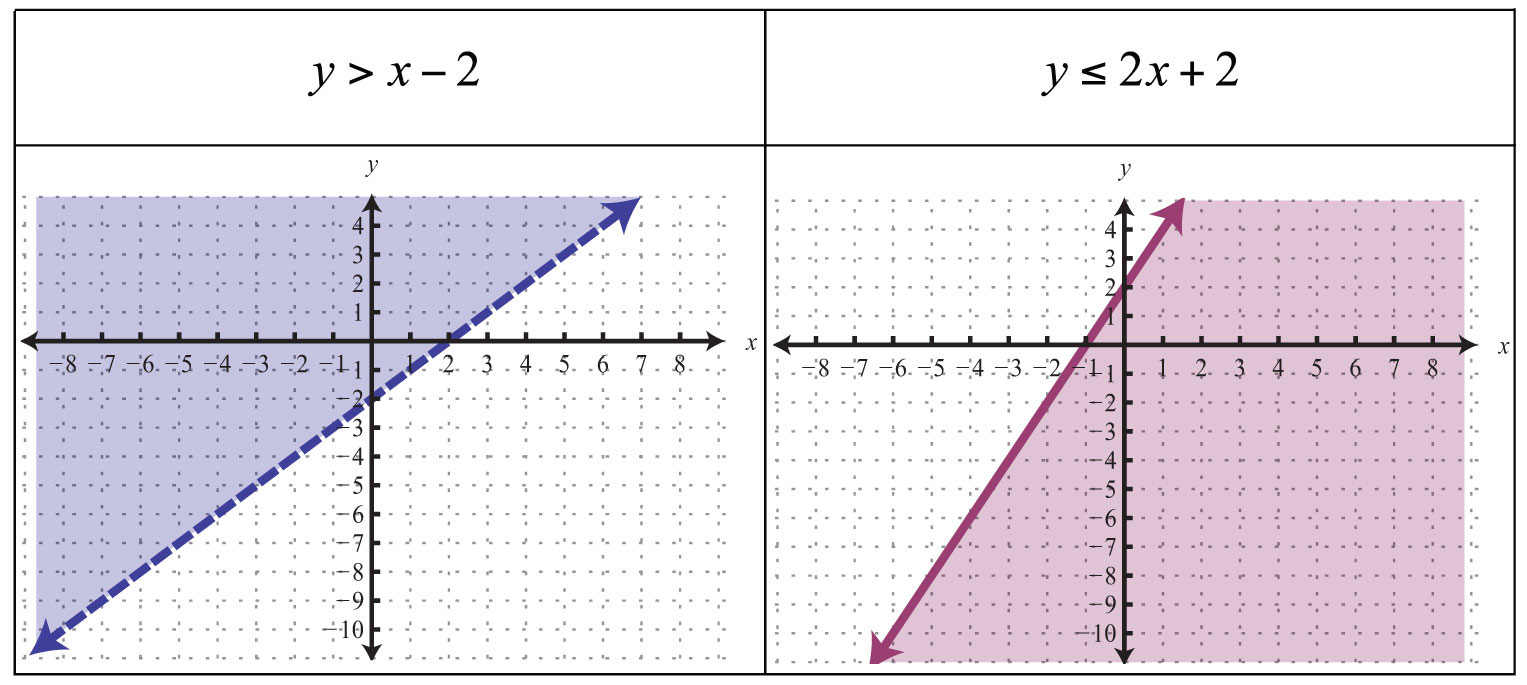06/15/2016 Graphing inequalities

Graphing InequalitiesSummer School Week 1 Review ( 06/10/2016)

RC3:A.5A

Solve linear equations in one variable, including those for which the application of the distributive property is necessary and for which variables are included on both sides.

Example:

Practice

RC3:A.2A

Determine the domain and range of a linear function in mathematical problems; determine reasonable domain and range values for real-world situations, both continuous and discrete; and represent domain and range using inequalities.

Example:

Practice

Rc3:A.3B

Calculate the rate of change of a linear function represented tabularly, graphically, or algebraically in context of mathematical and real-world problems.

Example:

Practice

RC3:A.3C

Graph linear functions on the coordinate plane and identify key features, including x-intercept, y-intercept, zeros, and slope, in mathematical and real-world problems

Example:

Practice

Quiz

Linear Functions Review RC3:A.2BGeometry: Intersecting Chords 04/26/2016On your notebook, work  out the solutions to the problems. No Work No Grade

Intersecting chords